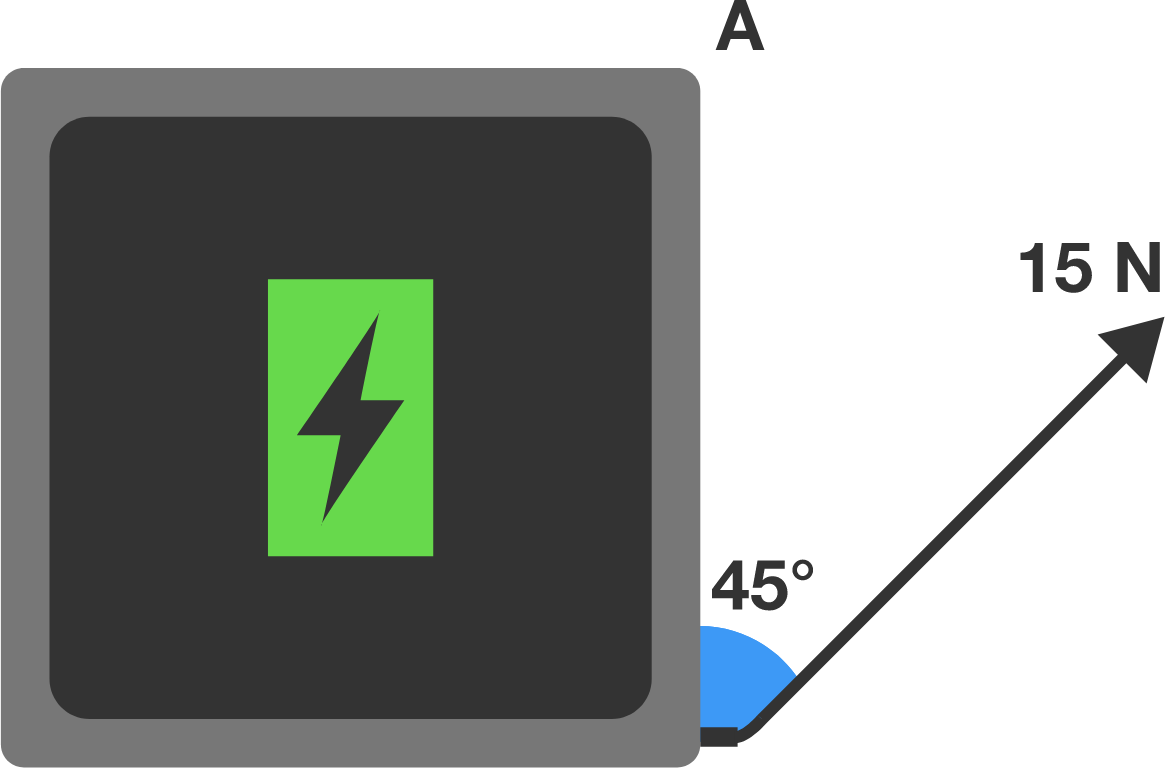Top view

A $1 \text{ kg}$ tablet with dimensions $30 \text{ cm} \times 30 \text{ cm}$ sits charging on a smooth table. The charging cord is attached to one of its corners. Somebody trips on the cord, applying a force of $15 \text{ N}$ to the tablet in the direction shown.

What is the initial acceleration at point $A$ in $\text{m/s}^2?$

Assume that tablet has uniform mass.

×